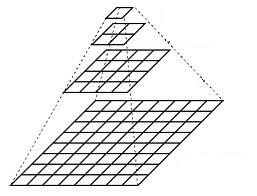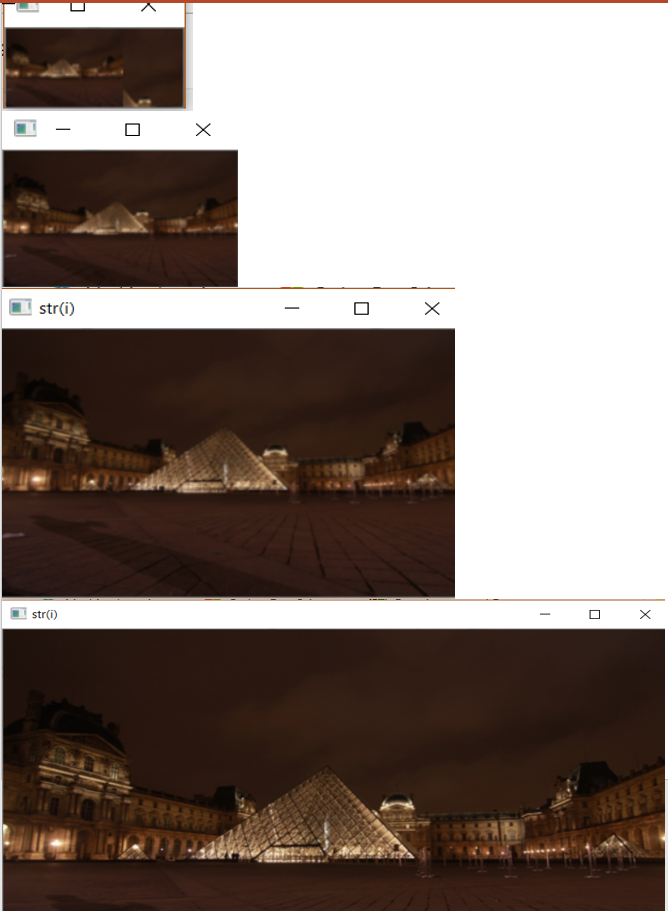Related Articles

# Image Pyramid using OpenCV | Python

• Last Updated : 20 May, 2019

Image Pyramids are one of the most beautiful concept of image processing.Normally, we work with images with default resolution but many times we need to change the resolution (lower it) or resize the original image in that case image pyramids comes handy.

The `pyrUp()` function increases the size to double of its original size and `pyrDown()` function decreases the size to half. If we keep the original image as a base image and go on applying `pyrDown` function on it and keep the images in a vertical stack, it will look like a pyramid. The same is true for upscaling the original image by `pyrUp` function.Once we scale down and if we rescale it to the original size, we lose some information and the resolution of the new image is much lower than the original one.

Below is an example of Image Pyramiding –

 `import` `cv2``import` `matplotlib.pyplot as plt`` ` `img ``=` `cv2.imread(``"images/input.jpg"``)`` ` `layer ``=` `img.copy()`` ` `for` `i ``in` `range``(``4``):``    ``plt.subplot(``2``, ``2``, i ``+` `1``)`` ` `    ``# using pyrDown() function``    ``layer ``=` `cv2.pyrDown(layer)`` ` `    ``plt.imshow(layer)``    ``cv2.imshow(``"str(i)"``, layer)``    ``cv2.waitKey(``0``)``     ` ` ` `cv2.destroyAllWindows()`

Output: